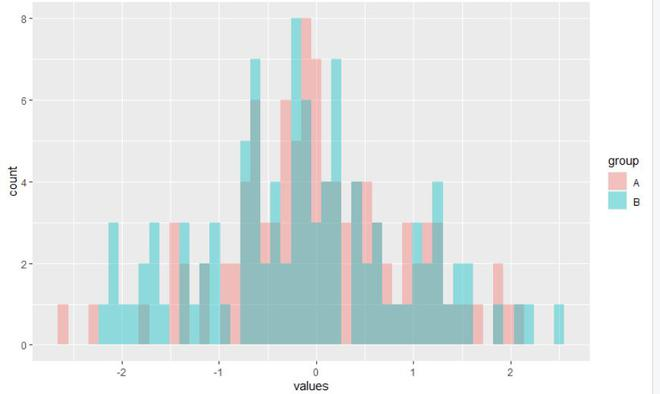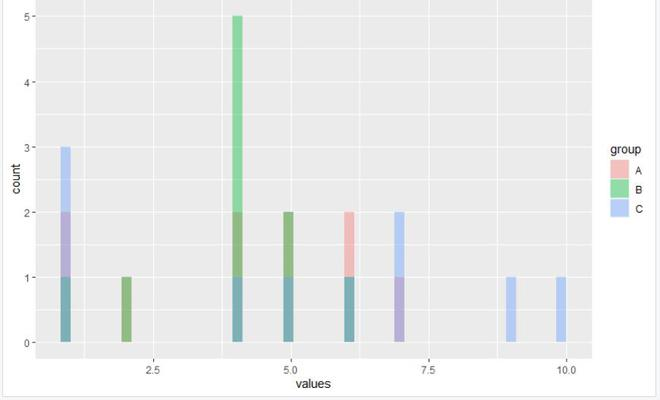# Draw Multiple Overlaid Histograms with ggplot2 Package in R

• Last Updated : 17 Jun, 2021

In this article, we are going to see how to draw multiple overlaid histograms with the ggplot2 package in the R programming language.

We will be drawing multiple overlaid histograms using the alpha argument of the geom_histogram() function from ggplot2 package. In this approach for drawing multiple overlaid histograms, the user first needs to install and import the ggplot2 package on the R console and call the geaom_histogram function with specifying the alpha argument of this function to a float value between 0 to 1 which will lead to the transparency of the different histogram plots on the same plot with the set of the data-frame as this function parameter to get multiple overlaid histograms in the R programming language.

geom_histogram() function: This function is an in-built function of ggplot2 module.

Syntax: geom_histogram(mapping = NULL, data = NULL, stat = “bin”, position = “stack”, …)

Parameters:

• mapping: The aesthetic mapping, usually constructed with aes or aes_string. Only needs to be set at the layer level if you are overriding the plot defaults.
• data: A layer-specific dataset – only needed if you want to override the plot defaults.
• stat: The statistical transformation to use on the data for this layer.
• position: The position adjustment to use for overlapping points on this layer

To install and import the ggplot2 package in the R console, the user needs to follow the following syntax:

```install.packages("ggplot2")
library("ggplot2")```

The alpha argument: This is a graphical parameter is a number from 0 to 1 opaque to transparent, it adjusts the transparency of the plot.

Example 1:

In this example, we will be taking 2 different 100 random data set to create 2 different histograms on the single plot using the alpha argument of the geom_histogram() function from the ggplot2 package in the R programming language.

## R

 `library``(``"ggplot2"``)``data <- ``data.frame``(values = ``c``(``rnorm``(100),``                              ``rnorm``(100)),``                   ``group = ``c``(``rep``(``"A"``, 100),``                             ``rep``(``"B"``, 100)))`` ` `ggplot``(data, ``aes``(x = values, fill = group)) +``geom_histogram``(position = ``"identity"``, alpha = 0.4, bins = 50)`

Output:Example 2:

In this example, we will be taking 3 different data to create 3 different histograms on a single plot using the alpha argument of the geom_histogram() function from the ggplot2 package in the R programming language.

## R

 `library``(``"ggplot2"``)``data <- ``data.frame``(values = ``c``(``c``(6,2,5,4,1,6,1,5,4,7),``                              ``c``(4,1,4,4,5,5,4,6,2,4),``                              ``c``(9,1,5,7,1,10,6,4,1,7)),``                   ``group = ``c``(``rep``(``"A"``, 10),``                             ``rep``(``"B"``, 10),``                             ``rep``(``"C"``, 10)))``ggplot``(data, ``aes``(x = values, fill = group)) +``geom_histogram``(position = ``"identity"``, alpha = 0.4, bins = 50)`

Output:My Personal Notes arrow_drop_up